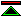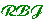# Truth by Convention

## by Willard Van Orman Quine

The paper consists of just three numbered but untitled sections, preceded by one introductory paragraph. The extra structure shown here is my analysis. In the text, very roughly, plain text is description, italicised text is discussion.

 Introduction Aim is to question the sense of the analytic/"non-conventional" distinction. I Truth arising from definitional conventions. II Truth arising from conventional postulations. III

### Introduction

While the doctrines of the physical sciences are generally recognised as "destined to retain always a non-conventional core", there is a widespread conviction "that logic and mathematics are purely analytic or conventional". Quine's purpose is to question the sense of this contrast.

So this is not just about "truth by convention" (which Quine seems to take as synonymous with "analyticity"), but an examination of the idea that mathematics is and science is not analytic. One may sense that Quine is hostile to the distinction, but he is cautious, perhaps even devious.

We can find here in the first paragraph of the essay, the seeds of the fallacious conclusions which follow. Quine here represents analyticity as arising from an increase in rigour accomplished by the introduction of piecemeal definitions of terms previously not defined. This he describes as the reconstrual of "theories about the world" as conventions of language.

Theories about the world, cannot be "reconstrued" as conventions. A contingent proposition is always a contingent proposition. Quine contrives to make the linguistic theory of necessity appear arbitrarily to classify facts as conventions, and hence to violate any genuine notion of necessity. Introducing a definition does not make any change to the logical status of sentences unless it changes their meaning, in which case the sentences no longer express the same proposition. What is arbitrary (if we chose to allow it as such) is the semantics of the languages we use, and these choices affect the connection between sentences and propositions, but not the status of the propositions themselves.

## I

A definition is "strictly, a convention of notational abbreviation".

"a definition is not a premise to a theory", truths involving defined terms are abbreviations of truths not involving those terms.

However, "logical consequences of definitions" are nevertheless "themselves truths of logic".

Definitions are not wholly arbitrary, "we have in mind some traditional usage of the sign antecedent to the definition", and the definition is not "satisfactory" unless it conforms to that traditional usage.

The notion of occurring vacuously and that of vacuous variant are defined (without being related to any antecedent tradition). Grammatically correct substitutions for a term occurring vacuously do not affecting the truth value of the enclosing sentence. A vacuous variant of a sentence is one in which some vacuously occurring term has been changed. A term whose occurrences are not all vacuous occurs essentially.

Quine argues that "if all mathematical expressions be defined in terms of logic, all truths involving only mathematical and logical expressions essentially become definitional abbreviations of truths of logic". This still applies where non-mathematical terms appear vacuously in a mathematical truth.

The idea that mathematical truths are elliptical for conditionals is discussed. This does render mathematics a part of logic, but in the same manner any science can be rendered a part of logic. "To incorporate mathematics into logic by regarding all recalcitrant mathematical truths as elliptical hypothetical statements is thus in effect merely to restrict the term mathematics to exclude those recalcitrant branches."

"It would thus appear that for the thesis that mathematics is logic it is not only sufficient but necessary that all mathematical expressions be capable of definition on the basis solely of logical ones."

Next Quine concedes that the same effect as regarding mathematical statements as elliptical for conditionals is achievable through contextual definitions, glosses over the fact that this method also admits theoretical science as logic, but claims that this fails because it misconstrues the truth values of certain empirical claims (specifically that a baseball is roughly a sphere).

Having asserted that definitions must be judged in part by their effects on empirical propositions, Quine goes on to assert that getting this right involves mathematical genius of a high order but concedes that: "the thesis that all mathematics reduces to logic is, however, substantiated by Principia to a degree satisfactory to most of us". But backs off with "There is no need here to adopt a final stand in this matter".

If this is allowed then mathematical truths become conventional transcriptions of logical truths. But mathematics is then not true by convention unless logic is.

Definitions suffice only to reduce mathematics to logic, they will not give us a basis for logical truth itself.

## II

In this section Quine considers whether logic is "true by convention". He discusses how conventions can be framed for the logical primitives. He seems to assume that this should be done by choosing truth values for sentences essentially involving the primitives. He notes that this would involve an infinite list of true sentences, and shows how such a list can be defined by rules which in effect amount to a deductive system. Though the example he works through conforms to ordinary usage of the primitives, he is at pains to point out that the choices involved are arbitrary, even to the extent that the supposed constraint of consistency need not be observed. He explicitly admits that preconceptions about the usage of the primitives may influence choices, but denies that they "enter into the rules of the game" (i.e. that the conventions are constrained by a need for consistency with prior usage).

## III

Having apparently conceded that logic and mathematics are "true by convention", meaning by this that they can be rendered so by adopting appropriate conventions about the truth of sentences of logic and mathematics, Quine now goes on to point out that the same can be said of the sentences of empirical science. Having apparently destroyed the pretentions of the idea of conventional truth to discriminate between logic and mathematics on the one hand, he then briefly considers the suggestion that the distinction is to be found in the idea that the former are a priori and the latter a posteriori. In discussing this he raises the further problem that the following of conventions formulated a rules presupposes a sufficient part of logic to enable the consequences of the rules to be establishes, and hence taints this account of logical truth with a problem of regress:
"if logic is to proceed mediately from conventions, logic is needed for inferring logic from the conventions"

1©created 2001/6/1 modified 2010/03/28